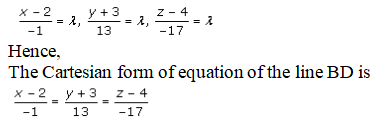# RD Sharma Solutions Class 12 Straight Line In Space Exercise 28.1

RD Sharma Solutions for Class 12 Maths Chapter 28 Straight Line in Space Exercise 28.1, is provided here. BYJU’S tutor team has prepared solutions which help students attain good marks in Maths. From the exam point of view, the solutions are solved in a simple and structured manner where students can secure an excellent score in the board exams. RD Sharma Solutions pdf can be downloaded from the links given below.

## Download PDF of Rd Sharma Solution for Class 12 Maths Chapter 28 Exercise 1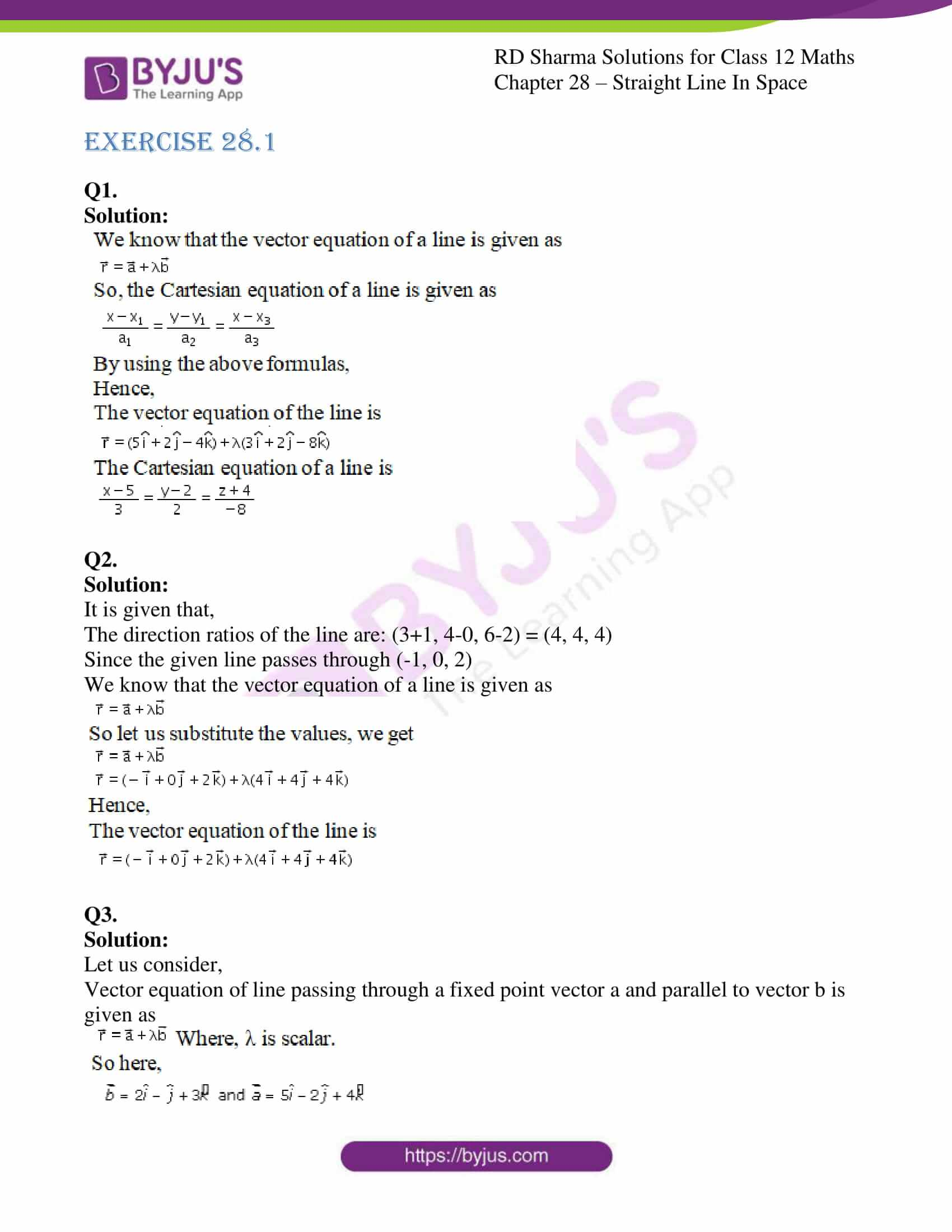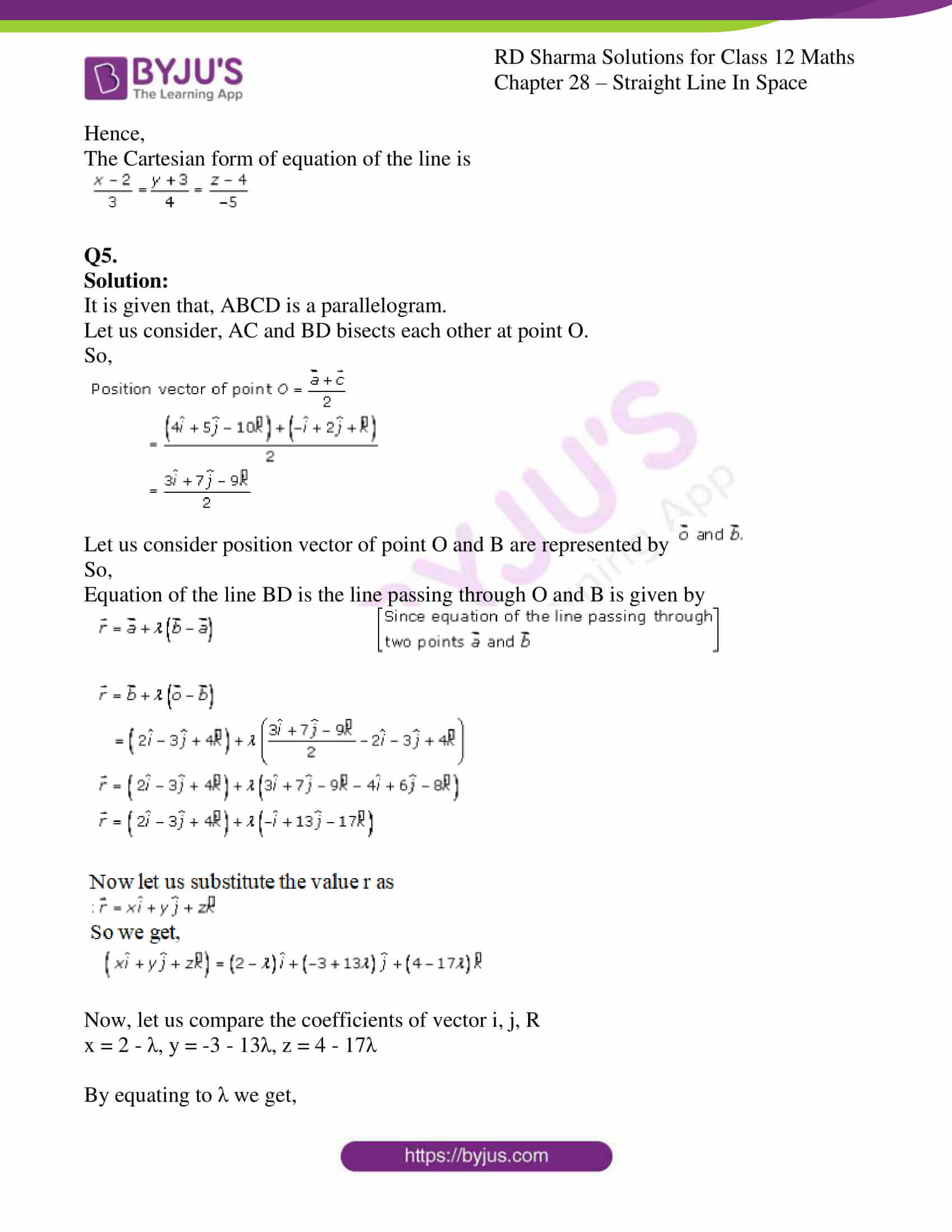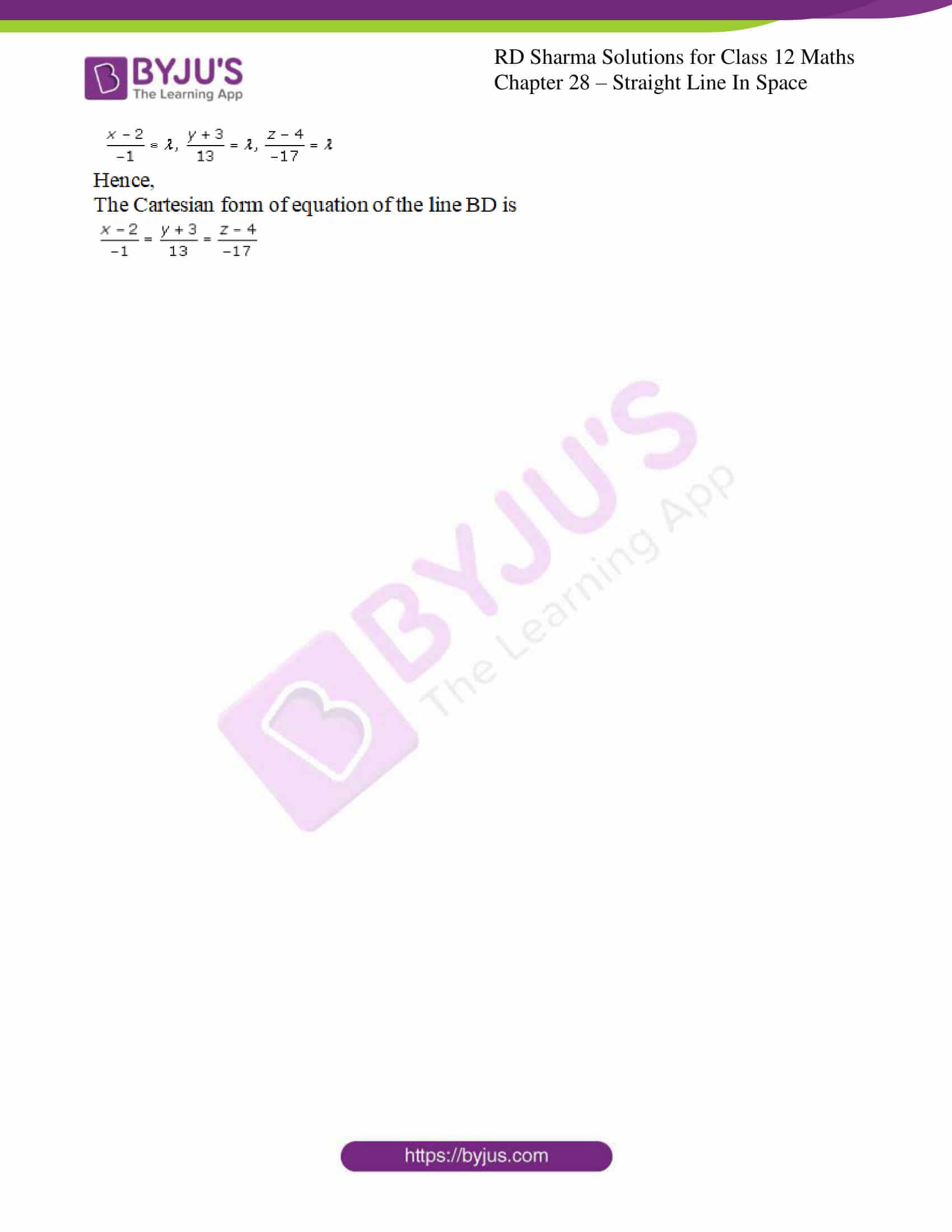### Access Answers for Rd Sharma Solution Class 12 Maths Chapter 28 Exercise 1

Q1.

Solution: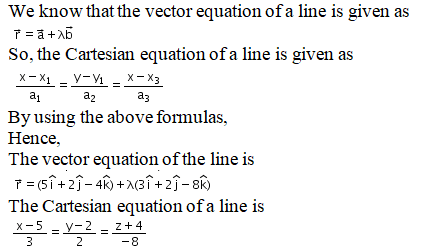Q2.

Solution:

It is given that,

The direction ratios of the line are: (3+1, 4-0, 6-2) = (4, 4, 4)

Since the given line passes through (-1, 0, 2)

We know that the vector equation of a line is given as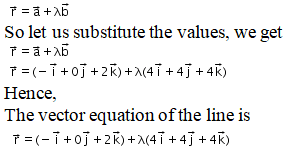Q3.

Solution:

Let us consider,

Vector equation of line passing through a fixed point vector a and parallel to vector b is given as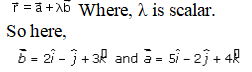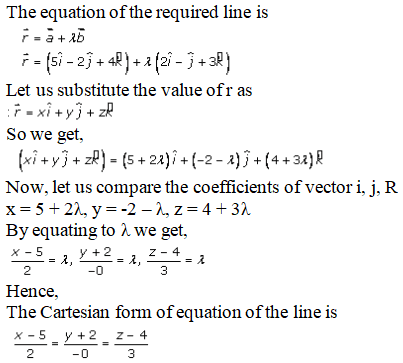Q4.

Solution:

Let us consider,

Vector equation of line passing through a fixed point vector a and parallel to vector b is given as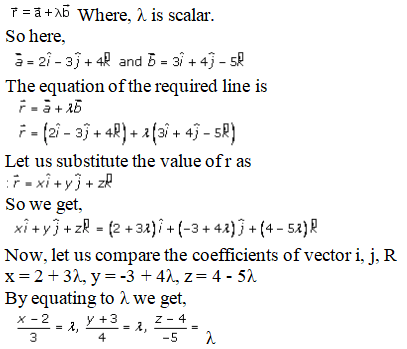Hence,

The Cartesian form of equation of the line is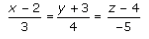Q5.

Solution:

It is given that, ABCD is a parallelogram.

Let us consider, AC and BD bisects each other at point O.

So,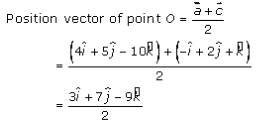Let us consider position vector of point O and B are represented by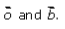So,

Equation of the line BD is the line passing through O and B is given by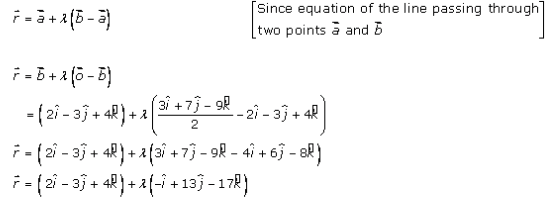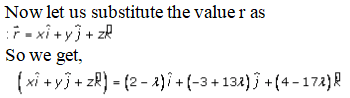Now, let us compare the coefficients of vector i, j, R

x = 2 – λ, y = -3 – 13λ, z = 4 – 17λ

By equating to λ we get,